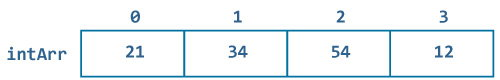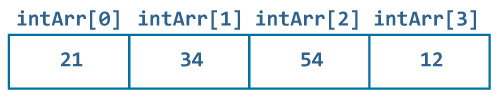Swift Arrays

# Swift Arrays

#### In this tutorial, you will learn about arrays, creating it, accessing values of an array and some common operations in array.

In the previous Swift Data Types article, we learned about creating variables/constants of some Data Type that can hold a single value.

But, what if we want to store multiple values of the same data type. We use something called Array in Swift.

## What is an array?

An array is simply a container that can hold multiple data (values) of a data type in an ordered list, i.e. you get the elements in the same order as you defined the items in the array.

An array can store values of any data type e.g.`Int`, `String`, class etc.

## How to declare an array in Swift?

You can create an empty array by specifying the data type inside square brackets `[]`.

Remember, You have to include the the type inside square brackets, otherwise Swift will treat it as a normal data type and you can store only a single value in it.

### Example 1: Declaring an empty array

``````let emptyIntArr:[Int] = []
print(emptyIntArr)
``````

When you run the program, the output will be:

`[ ]`

In the above program, we have declared a constant emptyIntArr that can store array of integer and initialized with 0 values.

OR

You can also define an empty array as below:

``````let emptyIntArr:Array<Int> = Array()
print(emptyIntArr)
``````

OR

Since, swift is a type inference language, you can also create array directly without specifying the Data Type but must initialize with some values so that compiler can infer its type as:

### Example 2: Declaring an array with some values

``````let someIntArr = [1, 2, 3, 4, 5, 6, 7, 8, 9]
print(someIntArr)
``````

When you run the program, the output will be:

`[1, 2, 3, 4, 5, 6, 7, 8, 9]`

In the above program, we have declared a constant someIntArr that can store array of Integer without specifying the type explicitly. Also, we have initialized array with 1, 2, 3, 4, 5, 6, 7, 8, 9 values.

### Example 3: Declaring an array containing the specified number of a single repeated value

You can also repeat a value a given number of times to form an array in Swift. This is done by using the array initializer with repeating and count.

``````let arrWithRepeatingValues = Array(repeating: "Hello, World", count: 4)
print(arrWithRepeatingValues)
``````

When you run the program, the output will be:

`["Hello, World", "Hello, World", "Hello, World", "Hello, World"]`

In the above program, we have defined a constant arrWithRepeatingValues that stores an array of string Hello, World and repeats the same value for 4 times as specified in the count.

Note: In Swift, you cannot create array of fixed-length size as you do in other programming languages. Fixed length size array means, array cannot have more elements than you define during initialization.

## How values are stored in array?

Suppose you have a constant that can store array of strings as below:

`let intArr = [21, 34, 54, 12]`

The pictorial representation of how values are stored in array can be shown below:All arrays you create starts with the index 0. The first element is stored in the index 0 , second element in the next index (1) and so on.

## How to access array elements in Swift?

You can access elements of an array by using subscript syntax, i.e.You need to include index of the value you want to access within square brackets immediately after the name of the array.

Suppose you declared an array intArr as above. The first element is intArr, second element is intArr and so on.### Example 4: Accessing elements of an array

``````let intArr = [21, 34, 54, 12]
print(intArr)
print(intArr)
print(intArr)
print(intArr)
``````

When you run the program, the output will be:

```21
34
54
12
```

You can also access elements of an array by using for-in loops. See Swift For-in loop to learn more about it.

## How to modify/add array elements in Swift?

You can modify elements of an array by using subscript syntax and assignment operator, i.e. you need to include index of the value you want to update within square brackets after the name of the array followed by the assignment operator and new value .

### Example 5: Modifying elements of an array

``````var intArr = [21, 34, 54, 12]
intArr = 12
intArr = 42
intArr = 45
intArr = 21
print(intArr)
``````

When you run the program, the output will be:

`[12, 42, 45, 21]`

You can also modify all the elements of array with new values as below:

### Example 6: Modifying an array as a whole

``````var intArr = [21, 34, 54, 12]
intArr = [1,2,3]
print(intArr)
``````

When you run the program, the output will be:

`[1, 2, 3]`

However, to add a new element to an existing array, you cannot use the subscript syntax. If you do, you'll end up with an error. You cannot do something like this:

### Example 7: Adding a new element in an array using subscript syntax (Doesn't work)

``````var intArr = [21, 34, 54, 12]
intArr = 10
``````

When you run the program, the output will be:

`fatal error: Index out of range`

The above program gives an error while assigning a new element to an array intArr. This is because, intArr has not allocated extra memory for the index 4 and cannot store the given value.

To correctly insert a new element to an array, we use array's `append()` method. `append()` is described in the below section.

## Some helpful built in Array functions & properties

### 1. isEmpty

This property determines if an array is empty or not. It returns `true` if an array does not contain any value otherwise returns `false`.

#### Example 8: How isEmpty works?

``````let intArr = [21, 34, 54, 12]
print(intArr.isEmpty)
``````

When you run the program, the output will be:

`false`

### 2. first

This property is used to access first element of an array.

#### Example 9: How first works?

``````let intArr = [21, 34, 54, 12]
print(intArr.first)
``````

When you run the program, the output will be:

`Optional(21)`

Similarly, you can use `last` property to access the last element of an array.

### 3. append

The append function is used to insert/append element at the end of the array.

#### Example 10: How append works?

``````var intArr = [21, 34, 54, 12]
intArr.append(32)
print(intArr)
``````

When you run the program, the output will be:

`[21, 34, 54, 12, 32]`

You can also append contents of one array to another array as:

``````var firstArr = [1,2,3,4]
var secondArr = [5,6,7,8]
firstArr.append(contentsOf: secondArr)
print(firstArr)
``````

When you run the program, the output will be:

`[1, 2, 3, 4, 5, 6, 7, 8]`

### 4. insert

This function is used to insert/append element at specific index of the array.

#### Example 11: How insert works?

``````var intArr = [21,34,54,12]
intArr.insert(22, at: 1)
print(intArr)
``````

When you run the program, the output will be:

`[21, 22, 34, 54, 12]`

Similarly you can also use `remove` property to remove element at specified index.

### 5. remove

This function removes and returns the value specified at the specified position from the array.

#### Example 12: How remove works?

``````var strArr = ["ab","bc","cd","de"]
let removedVal = strArr.remove(at: 1)
print("removed value is \(removedVal)")
print(strArr)
``````

When you run the program, the output will be:

```removed value is bc
["ab", "cd", "de"]
```

Similarly, you can also use functions like `removeFirst` to remove first element of an array, `removeLast` to remove last element of an array and `removeAll` to empty an array.

### 6. reversed

This function returns the elements of array in reverse order.

#### Example 13: How reversed() works?

``````var intArr = [21,22,23,24]
let reversedArr = Array(intArr.reversed())
print(reversedArr)
``````

When you run the program, the output will be:

`[24, 23, 22, 21]`

### 7. count

This property returns the total number of elements in an array.

#### Example 14: count

``````let floatArr = [10.2,21.3,32.0,41.3]
print(floatArr.count)
``````

When you run the program, the output will be:

`4`

## Things to Remember

While using subscript syntax to access elements of an array in Swift, you must be sure the value lies in the index otherwise you will get a runtime crash. Let's see this in example:

``````let intArr = [21, 34, 54, 12]
print(intArr[-1])
``````

When you run the program, the output will be:

`fatal error: Index out of range`

In the above program, there is no value in the index -1. So when you try to access the value in the index you will get a runtime crash.

To prevent this, first find the index of the element you are trying to remove. And then remove element at the index as below:

``````var intArr = [21, 34, 54, 12]
if let index = intArr.index(of: 34) {
print("found index")
let val =  intArr.remove(at: index)
print(val)
}
``````

When you run the program, the output will be:

```found index
34
```